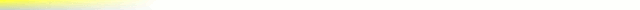# [mkgmap-dev] Trouble getting some multipolygons to render inmkgmap

From Torsten Leistikow de_muur at gmx.de on Mon Sep 27 15:18:18 BST 2010

```charlie at cferrero.net schrieb am 27.09.2010 15:20:
>> As an example take a nature reserve consisting of a wood with a lake inside.
>> This migth be mapped with two polygons and a relation:
>> polygon A: leisure=nature_reserve (the complete area)
>> polygon B: natural=water (only the inner area)
>> multipolygon relation: natural=wood and outer=polygon A and inner=polygon B
>> (only the surrounding area)
>>
>> Right now polygon A seems to be missing in the resulting map.
>>
> But how would mkgmap know which of the two outer polygon types to use
> (ie nature reserve or wood)?

It should use both:

The nature reserve should cover the complete area.

The wood should cover only the area defined by the multipolygon.

This is (one of) the intended tagging of the multipolygons. Allowed alternatives
(with the same logical interpretation) would be:

1. You could use an additional polygon for the outer limit of the multipolygon
(polygon C) which would have the same nodes as polygon A. Polygon A and B would
stay unchanged.
multipolygon relation: natural=wood and outer=polygon C and inner=polygon B

2. You could put all tags from the relation on polygon C, polygon A and B would
stay unchanged.
polygon B: natural=wood
multipolygon relation: outer=polygon A and inner=polygon B

3. You could move the nature reserve tag into the multipolygon area and the
inner area.
polygon A:
polygon B: natural=water and leisure=nature_reserve
multipolygon relation: natural=wood and leisure=nature_reserve and outer=polygon
A and inner=polygon B

4. And you could move the tags from the relation of variant 3 to the outer polygon.
polygon A: natural=wood and leisure=nature_reserve
polygon B: natural=water and leisure=nature_reserve
multipolygon relation: outer=polygon A and inner=polygon B

I think these five possibilities are all allowed under the actual accepted
multipolygon scheme and they should all result in nearly the same garmin map.
(Alternative 3 and 4 split the nature reserve into to areas, but in the end it
covers teh same area).

Gruss
Torsten

```